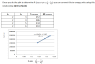# How do I calculate Energy?

• whatisphysics

## Homework Statement

I made a word doc, and attached it as a picture.

## The Attempt at a Solution

I calculated all the wavelengths, and plotted the values, but how do I find energy from the equation deltaE = hv?

#### Attachments

•chem3.PNG
7.4 KB · Views: 471
Last edited:
Looks to me like just a plug and chug, I am not sure what's the problem.

Looks to me like just a plug and chug, I am not sure what's the problem.

But what is the R, and what is the c?

thanks.

R is what you are to find from the plot (so called Rydberg constant), c is the speed of light.

R2 mentioned on the plot seems to be a correlation coefficient, this can be confusing.